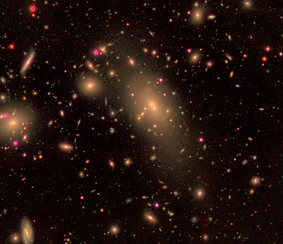top of page

# DR7 SDSS CATALOGS

###### Click here for the NYU-VAGC directory corresponding to the dr72bright34 files.## sdss_kdgroups_v1.0.dat

This is an ascii file with 559038 lines. The file is line-by-line parallel with the NYU VAGC files for the dr72bright34 set of files. The fields in the file are:

1. id [int] - 0-indexed list of galaxies. This values should go in order from 0 to NGAL-1.

2. RA [degrees; float] - right ascension of the galaxy

3. Dec [degrees; float] - decliration of the galaxy

4. z [float] - redshift of the galaxy

5. L_gal [L_sol/h^-2; float] - r-band luminosity of the galaxy in solar units, assuming M_r,sol=4.65 and h=1.

6. V_max [(Mpc/h)^3; float] - maximum volume at which each galaxy can be observed in SDSS. Taken from NYU VAGC

7. P_sat [float] - probability that this galaxy is a satellite. If P_sat>0.5, it is assumed to be a satellite and will be assigned to a group with a different central galaxy.

8. M_halo [M_sol/h; float] - Halo mass that the galaxy is assigned to. If the galaxy is a satellite, this is the parent halo's mass.

9. N_sat [float] - If the galaxy is a central (P_sat<0.5), this is the number of satellites within that halo. This is a float rather than an int becuase, for volume-limited samples, this number is corrected for redshift boundaries using an error function.

10. L_tot [L_sol/h^2; float] - The total luminosity of the group that the galaxy occupies.

11. igrp [int] - The id of the central galaxy for the group (column 1). If the galaxy is a central, igrp=id. This is also the 0-indexed line of the file.

## sdss_fluxlim_v1.0.datThis is an ascii file with 559038 lines. The file is line-by-line parallel with the NYU VAGC files for the dr72bright34 set of files. This file is the input for the group finder results linked above. The columns are:

1. RA [degrees; float] - right ascension of the galaxy

2. Dec [degrees; float] - decliration of the galaxy

3. z [float] - redshift of the galaxy

4. log L_gal [L_sol/h^-2; float] - r-band luminosity of the galaxy in solar units, assuming M_r,sol=4.65 and h=1.

5. V_max [(Mpc/h)^3; float] - maximum volume at which each galaxy can be observed in SDSS. Taken from NYU VAGC

6. color_flag [int]- 1=quiescent, 0=star-forming. This is based on the GMM cut of the Dn4000 data in Tinker 2020.

7. chi [dimensionless] - THis is the normalized galaxy concentration. See details in both papers.## sdss_galprops_v1.0.dat

This is an ascii file with 559038 lines. The file is line-by-line parallel with the NYU VAGC files for the dr72bright34 set of files. This file contains extra information about each galaxy that may be useful. For spectroscopically-derived quantities, if a galaxy is "collided" the quantity is drawn randomly from it's nearest neighbors in color-magnitude space. The columns are:

1. Mag_g [magnitudes; float] - k-corrected and evolution corrected (to z=0.1) g-band absolute magnitude.

2. Mag_r [magnitudes; float] - k-corrected and evolution corrected (to z=0.1) r-band absolute magnitude.

3. sigma_v [km/s; float] - Stellar velocity dispersion.

4. Dn4000 [no units, float] - Narrow 4000-Angstrom break. Taken from MPA-JHU DR7 catalog.

5. concentration [no units; float] - Galaxy concentration, c_90/c_50, using the Petrosian radii.

6. log M_star [M_sol; float] - log10 of the PCA-based galaxy stellar mass, assuming Maraston 2011 stellar population synthesis models. Assumes h=0.7.

bottom of page